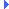FD.io VPP  v17.07-30-g839fa73 Vector Packet Processing
svm_fifo_segment.h File ReferenceInclude dependency graph for svm_fifo_segment.h:This graph shows which files directly or indirectly include this file:

Go to the source code of this file.

## Data Structures

struct  svm_fifo_segment_private_t

struct  svm_fifo_segment_main_t

struct  svm_fifo_segment_create_args_t

## Macros

#define foreach_ssvm_fifo_segment_api_error   _(OUT_OF_SPACE, "Out of space in segment", -200)

## Enumerations

enum  svm_fifo_segment_freelist_t { FIFO_SEGMENT_FREELIST_NONE = -1, FIFO_SEGMENT_RX_FREELIST = 0, FIFO_SEGMENT_TX_FREELIST, FIFO_SEGMENT_N_FREELISTS }

enum  ssvm_fifo_segment_api_error_enum_t { foreach_ssvm_fifo_segment_api_error }

## Functions

static svm_fifo_segment_private_tsvm_fifo_get_segment (u32 segment_index)

static u8 svm_fifo_segment_has_fifos (svm_fifo_segment_private_t *fifo_segment)

static svm_fifo_tsvm_fifo_segment_get_fifo_list (svm_fifo_segment_private_t *fifo_segment)

int svm_fifo_segment_create (svm_fifo_segment_create_args_t *a)
(master) create an svm fifo segment More...

int svm_fifo_segment_create_process_private (svm_fifo_segment_create_args_t *a)
Create an svm fifo segment in process-private memory. More...

int svm_fifo_segment_attach (svm_fifo_segment_create_args_t *a)
(slave) attach to an svm fifo segment More...

void svm_fifo_segment_delete (svm_fifo_segment_private_t *s)

svm_fifo_tsvm_fifo_segment_alloc_fifo (svm_fifo_segment_private_t *s, u32 data_size_in_bytes, svm_fifo_segment_freelist_t index)

void svm_fifo_segment_free_fifo (svm_fifo_segment_private_t *s, svm_fifo_t *f, svm_fifo_segment_freelist_t index)

void svm_fifo_segment_init (u64 baseva, u32 timeout_in_seconds)

u32 svm_fifo_segment_index (svm_fifo_segment_private_t *s)

## Variables

svm_fifo_segment_main_t svm_fifo_segment_main

## Macro Definition Documentation

 #define foreach_ssvm_fifo_segment_api_error   _(OUT_OF_SPACE, "Out of space in segment", -200)

Definition at line 85 of file svm_fifo_segment.h.

## Enumeration Type Documentation

Enumerator
foreach_ssvm_fifo_segment_api_error

Definition at line 88 of file svm_fifo_segment.h.

Enumerator
FIFO_SEGMENT_FREELIST_NONE
FIFO_SEGMENT_RX_FREELIST
FIFO_SEGMENT_TX_FREELIST
FIFO_SEGMENT_N_FREELISTS

Definition at line 22 of file svm_fifo_segment.h.

## Function Documentation

 static svm_fifo_segment_private_t* svm_fifo_get_segment ( u32 segment_index )
inlinestatic

Definition at line 67 of file svm_fifo_segment.h.Here is the caller graph for this function:

 svm_fifo_t* svm_fifo_segment_alloc_fifo ( svm_fifo_segment_private_t * s, u32 data_size_in_bytes, svm_fifo_segment_freelist_t index )

Definition at line 221 of file svm_fifo_segment.c.Here is the call graph for this function:Here is the caller graph for this function:

 int svm_fifo_segment_attach ( svm_fifo_segment_create_args_t * a )

(slave) attach to an svm fifo segment

Definition at line 178 of file svm_fifo_segment.c.Here is the call graph for this function:

 int svm_fifo_segment_create ( svm_fifo_segment_create_args_t * a )

(master) create an svm fifo segment

Definition at line 87 of file svm_fifo_segment.c.Here is the call graph for this function:Here is the caller graph for this function:

 int svm_fifo_segment_create_process_private ( svm_fifo_segment_create_args_t * a )

Create an svm fifo segment in process-private memory.

Definition at line 138 of file svm_fifo_segment.c.Here is the call graph for this function:Here is the caller graph for this function:

 void svm_fifo_segment_delete ( svm_fifo_segment_private_t * s )

Definition at line 213 of file svm_fifo_segment.c.Here is the call graph for this function:Here is the caller graph for this function:

 void svm_fifo_segment_free_fifo ( svm_fifo_segment_private_t * s, svm_fifo_t * f, svm_fifo_segment_freelist_t index )

Definition at line 289 of file svm_fifo_segment.c.Here is the call graph for this function:Here is the caller graph for this function:

 static svm_fifo_t* svm_fifo_segment_get_fifo_list ( svm_fifo_segment_private_t * fifo_segment )
inlinestatic

Definition at line 80 of file svm_fifo_segment.h.Here is the caller graph for this function:

 static u8 svm_fifo_segment_has_fifos ( svm_fifo_segment_private_t * fifo_segment )
inlinestatic

Definition at line 74 of file svm_fifo_segment.h.Here is the caller graph for this function:

 u32 svm_fifo_segment_index ( svm_fifo_segment_private_t * s )

Definition at line 341 of file svm_fifo_segment.c.

 void svm_fifo_segment_init ( u64 baseva, u32 timeout_in_seconds )

Definition at line 332 of file svm_fifo_segment.c.Here is the caller graph for this function:

## Variable Documentation

 svm_fifo_segment_main_t svm_fifo_segment_main

Definition at line 18 of file svm_fifo_segment.c.